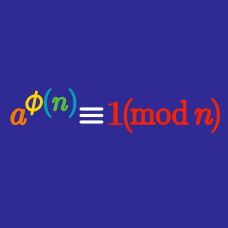Number Theory

# Diffie-Hellman

Solving which of the following problems would allow one to break the Diffie-Hellman protocol?

Suppose Alice and Bob choose $$p=191$$ and $$g=2$$. If Alice's secret number is $$12$$ and Bob's is $$16$$, what is the shared secret key?

Which of the following primes is most likely to be used in the Diffie-Hellman protocol?

Alice and Bob unwisely choose $$p=211$$ for their Diffie-Hellman protocol, along with $$g=2$$. Eve sees the transmission $$g^n \pmod p = 155$$ and the transmission $$g^m \pmod p = 96$$. What is the shared secret key $$g^{mn} \pmod p$$?

Which of the following modifications would NOT increase the security of the Diffie-Hellman protocol?

×

Problem Loading...

Note Loading...

Set Loading...